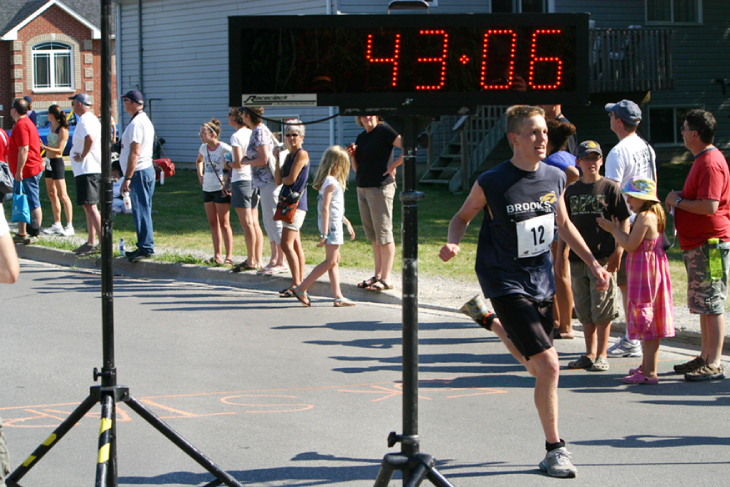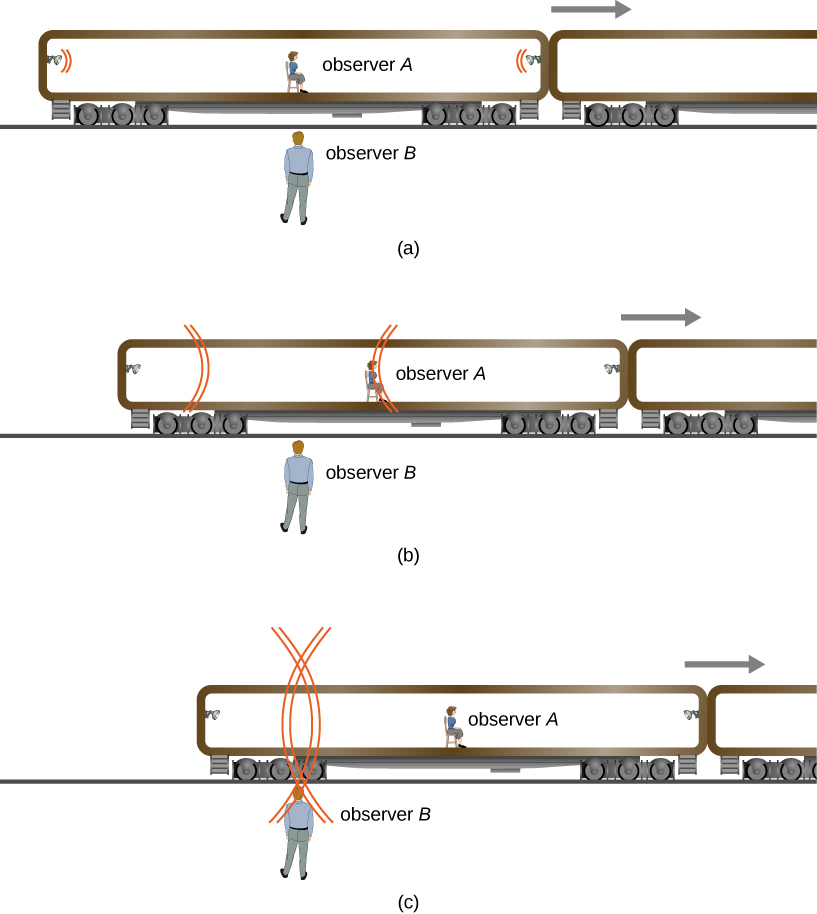# 5.2 Relativity of simultaneity

 Page 1 / 1
By the end of this section, you will be able to:
• Show from Einstein's postulates that two events measured as simultaneous in one inertial frame are not necessarily simultaneous in all inertial frames.
• Describe how simultaneity is a relative concept for observers in different inertial frames in relative motion.

Do time intervals depend on who observes them? Intuitively, it seems that the time for a process, such as the elapsed time for a foot race ( [link] ), should be the same for all observers. In everyday experiences, disagreements over elapsed time have to do with the accuracy of measuring time. No one would be likely to argue that the actual time interval was different for the moving runner and for the stationary clock displayed. Carefully considering just how time is measured, however, shows that elapsed time does depends on the relative motion of an observer with respect to the process being measured.Elapsed time for a foot race is the same for all observers, but at relativistic speeds, elapsed time depends on the motion of the observer relative to the location where the process being timed occurs. (credit: "Jason Edward Scott Bain"/Flickr)

Consider how we measure elapsed time. If we use a stopwatch, for example, how do we know when to start and stop the watch? One method is to use the arrival of light from the event. For example, if you’re in a moving car and observe the light arriving from a traffic signal change from green to red, you know it’s time to step on the brake pedal. The timing is more accurate if some sort of electronic detection is used, avoiding human reaction times and other complications.

Now suppose two observers use this method to measure the time interval between two flashes of light from flash lamps that are a distance apart ( [link] ). An observer A is seated midway on a rail car with two flash lamps at opposite sides equidistant from her. A pulse of light is emitted from each flash lamp and moves toward observer A , shown in frame (a) of the figure. The rail car is moving rapidly in the direction indicated by the velocity vector in the diagram. An observer B standing on the platform is facing the rail car as it passes and observes both flashes of light reach him simultaneously, as shown in frame (c). He measures the distances from where he saw the pulses originate, finds them equal, and concludes that the pulses were emitted simultaneously.

However, because of Observer A ’s motion, the pulse from the right of the railcar, from the direction the car is moving, reaches her before the pulse from the left, as shown in frame (b). She also measures the distances from within her frame of reference, finds them equal, and concludes that the pulses were not emitted simultaneously.

The two observers reach conflicting conclusions about whether the two events at well-separated locations were simultaneous. Both frames of reference are valid, and both conclusions are valid. Whether two events at separate locations are simultaneous depends on the motion of the observer relative to the locations of the events.(a) Two pulses of light are emitted simultaneously relative to observer B . (c) The pulses reach observer B ’s position simultaneously. (b) Because of A ’s motion, she sees the pulse from the right first and concludes the bulbs did not flash simultaneously. Both conclusions are correct.

Here, the relative velocity between observers affects whether two events a distance apart are observed to be simultaneous. Simultaneity is not absolute . We might have guessed (incorrectly) that if light is emitted simultaneously, then two observers halfway between the sources would see the flashes simultaneously. But careful analysis shows this cannot be the case if the speed of light is the same in all inertial frames.

This type of thought experiment (in German, “Gedankenexperiment”) shows that seemingly obvious conclusions must be changed to agree with the postulates of relativity. The validity of thought experiments can only be determined by actual observation, and careful experiments have repeatedly confirmed Einstein’s theory of relativity.

## Summary

• Two events are defined to be simultaneous if an observer measures them as occurring at the same time (such as by receiving light from the events).
• Two events at locations a distance apart that are simultaneous for an observer at rest in one frame of reference are not necessarily simultaneous for an observer at rest in a different frame of reference.

#### Questions & Answers

A round diaphragm S with diameter of d = 0.05 is used as light source in Michelson interferometer shown on the picture. The diaphragm is illuminated by parallel beam of monochromatic light with wavelength of λ = 0.6 μm. The distances are A B = 30, A C = 10 . The interference picture is in the form of concentric circles and is observed on the screen placed in the focal plane of the lens. Estimate the number of interference rings m observed near the main diffractive maximum.
A Pb wire wound in a tight solenoid of diameter of 4.0 mm is cooled to a temperature of 5.0 K. The wire is connected in series with a 50-Ωresistor and a variable source of emf. As the emf is increased, what value does it have when the superconductivity of the wire is destroyed?
how does colour appear in thin films
in the wave equation y=Asin(kx-wt+¢) what does k and w stand for.
derivation of lateral shieft
hi
Imran
total binding energy of ionic crystal at equilibrium is
How does, ray of light coming form focus, behaves in concave mirror after refraction?
Refraction does not occur in concave mirror. If refraction occurs then I don't know about this.
Sushant
What is motion
Anything which changes itself with respect to time or surrounding
Sushant
good
Chemist
and what's time? is time everywhere same
Chemist
No
Sushant
how can u say that
Chemist
do u know about black hole
Chemist
Not so more
Sushant
DHEERAJ
These substance create harmful radiation like alpha particle radiation, beta particle radiation, gamma particle radiation
Sushant
But ask anything changes itself with respect to time or surrounding A Not any harmful radiation
DHEERAJ
explain cavendish experiment to determine the value of gravitational concept.
Cavendish Experiment to Measure Gravitational Constant. ... This experiment used a torsion balance device to attract lead balls together, measuring the torque on a wire and equating it to the gravitational force between the balls. Then by a complex derivation, the value of G was determined.
Triio
For the question about the scuba instructor's head above the pool, how did you arrive at this answer? What is the process?
as a free falling object increases speed what is happening to the acceleration
of course g is constant
Alwielland
acceleration also inc
Usman
which paper will be subjective and which one objective
jay
normal distributiin of errors report
Dennis
normal distribution of errors
Dennis
acceleration also increases
Jay
there are two correct answers depending on whether air resistance is considered. none of those answers have acceleration increasing.
Michael
Acceleration is the change in velocity over time, hence it's the derivative of the velocity with respect to time. So this case would depend on the velocity. More specifically the change in velocity in the system.
Big
photo electrons doesn't emmit when electrons are free to move on surface of metal why?
What would be the minimum work function of a metal have to be for visible light(400-700)nm to ejected photoelectrons?
give any fix value to wave length
Rafi
40 cm into change mm
40cm=40.0×10^-2m =400.0×10^-3m =400mm. that cap(^) I have used above is to the power.
Prema
i.e. 10to the power -2 in the first line and 10 to the power -3 in the the second line.
Prema
there is mistake in my first msg correction is 40cm=40.0×10^-2m =400.0×10^-3m =400mm. sorry for the mistake friends.
Prema
40cm=40.0×10^-2m =400.0×10^-3m =400mm.
Prema
this msg is out of mistake. sorry friends​.
Prema
what is physics?ByByByBy RhodesByByBy Rhodes